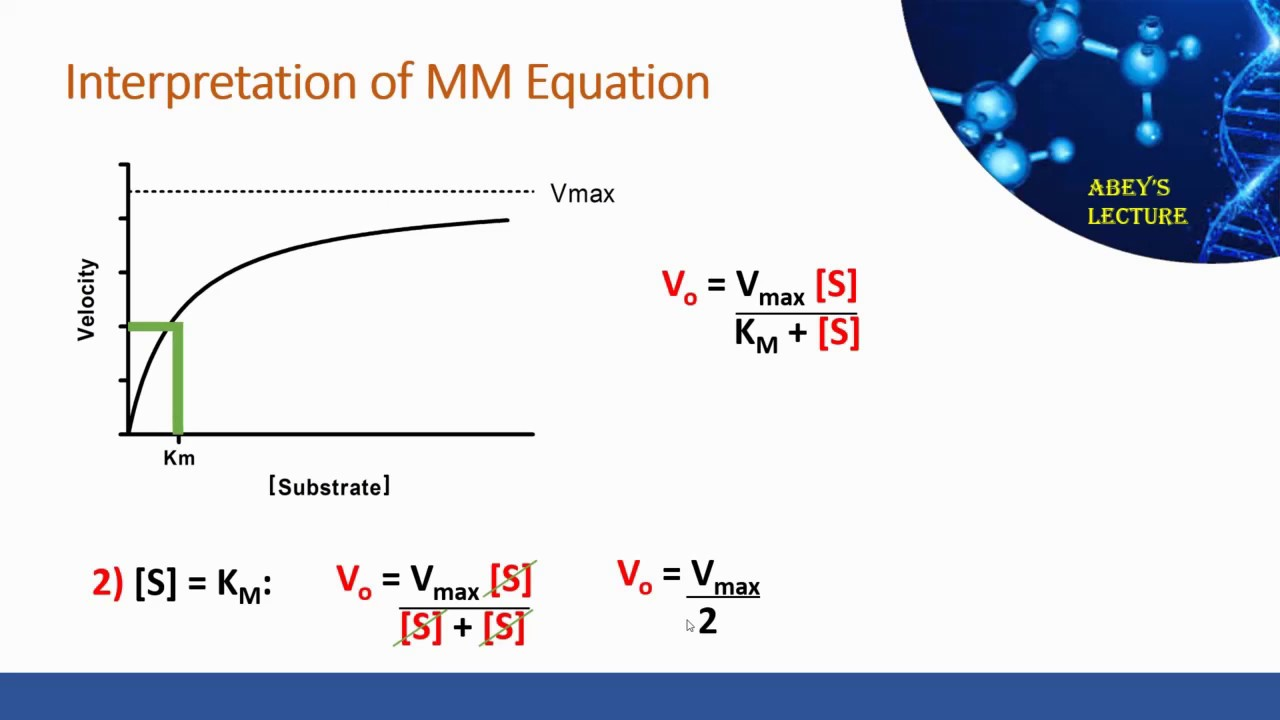# Enzyme Kinetics and Question Answer for NEET, GPAT, GATE, SSC, Pharmacist, CSIR NET, Nursing Exam

## Enzyme Kinetics and Question Answer for NEET, GPAT, GATE, SSC, Pharmacist, CSIR NET, Nursing Exam

Kinetics is the study of reaction rates and how they are changed in response to any environmental factors. The key factor which affects the rate of reaction catalyzed by an enzyme is the amount of substrate present [S], the effect of V0 (initial velocity).

• At comparatively low concentration of substrate, V0 increases linearly with an increase in substrate concentration [S]. this is known as 1st order kinetics.
• At high [S], the V0 increases by smaller and smaller amounts in response to the [S].
• Finally, the reaction reaches a point where there is only small increase in the initial velocity, with increasing [S]. this plateau state is called maximum velocity, Vmax .

In any enzyme catalyzed reaction, the enzyme exist in two forms: free or uncombined form E and combined form ES(enzyme-substrate complex).

• At low [S], enzyme remains in uncombined form E. In this rate of reaction is directly proportional to [S].
• The maximum velocity (Vmax) of an enzymatic reaction is observed when all the enzymes are present in ES form. In this condition, enzyme is saturated with its substrate. So any further increases in [S] will have no effect on the rate of reaction and the reaction achieves the steady state.

The ES complex breaks down and yields product and now the enzyme is free to bind to another substrate.

1. Michaelis-Menten Equation

Enzymatic reactions occur in two stages shown in below equations:

K1, K2 and K3 are rate constant. Michaelis-Menten Equation describes how reaction velocity varies substrate concentration. The mathematical equation is given as:-This picture is taken only for educational purpose from physiology web

Where,

• V0= initial reaction velocity
• Vmax= maximum velocity
• Km= Michaelis-Menten constant
• [S] = substrate concentration.1. Lineweaver-Burk plot or Double Reciprocal Plot

A more convenient procedure to find out the values for Km and Vmax is double-reciprocal plot. The equation is obtained by taking the reciprocal of Michaelis-Menten equation:

When 1/V is plotted against 1/[S], a straight line obtains with a slope equal to Km/Vmax. The point at which line intersects the Y-axis, is equal to 1/Vmax and the point at which line intersects the x-axis is equal to -1/Km.

## Multiple Choice Questions (MCQs)

1. What is enzyme kinetics?

A. Study of [S]

B. Study of rate of reaction

C. Study of initial velocity

D. All of the above

2. What is the effect on initial velocity if the substrate concentration is low?

A. Increases rapidly

B. Increases slowly

C. Decreases

D. Remains constant

3. What is the effect on initial velocity if the substrate concentration is high?

A. Increases rapidly

B. Increases slowly

C. Decreases

D. Remains constant

4. In how many forms, enzymes exists in an enzymatic reaction?

A. 2

B. 3

C. 1

D. 4

5. In which form the enzyme exists when the maximum velocity is observed?

A. E form

B. ES form

C. Both

D. None

6. In which form the enzyme exists when [S] is low?

A. E form

B. ES form

C. Both

D. None

7. When the reaction reaches its plateau state, which type of kinetics is seen?

A. Zero order kinetics

B. First order kinetics

C. Second order kinetics

D. None of the above

8. Match the following-

a. initial velocity                                                 1. [S]

b. Michaelis-Menten constant                         2. V0

c. maximum velocity                                          3. K(M)

d. substrate concentration                               4. V(MAX)

9. Which equation is required to determine the mechanism of action of enzyme inhibitors?

A. Michaelis-Menten Equation

B. Lineweaver-Burk plot

C. Double reciprocal plot

D. Both B and C

10. Turnover number is also known as?

A. Km

B. Kcat

C. Kcam

11. In which condition, V0=Vmax?

A. Low [S]

B. High [S]

C. Low V0

D. High V0

12. Which of the following statement is NOT true?

A. Turnover number is also known as catalytic constant

B. The reaction for the formation of ES is irreversible

C. The reaction is in steady state when rate of synthesis is equal to rate of degradation

D. Plateau is called Vmax

13. Which of the following is the slope of double-reciprocal plot?

A. Km/V0

B. Vmax/Km

C. -1/Km

D. None of the above

14. Which of the following terms are involved in Michaelis-Menten Equation?

A. Vmax

B. Km

C. V0

D. All of the above

15. The point at which the line intersects the y-axis of double-reciprocal plot is numerically equal to?

A. Km/V0

B. Vmax/Km

C. -1/Km

D. 1/Vmax

1. Study of rate of reaction

2. Increases rapidly

3. Increases slowly

4. 2

5. ES form

6. E form

7. Zero order kinetics

8. a – 2 b – 3 c – 4 d – 1

9. Both B and C

10. Kcat

11. Low [S]

12. The reaction for the formation of ES is irreversible

13. None of the above

14. All of the above

15. 1/Vmax

For More Standard and Quality Question Bank you can Join Our Test Series Programme for GPAT, NIPER JEE, Pharmacist Recruitment Exam, Drug Inspector Recruitment Exams, PhD Entrance Exam for Pharmacy

Participate in CSIR NET JRF Mock Test

Participate GATE Mock Test

REFERENCE:- Pankaja Naik- Biochemistry; 4th edition; page no:- 88-90.

×
Free Video Lectures of Pharmacy Exams
Apply now